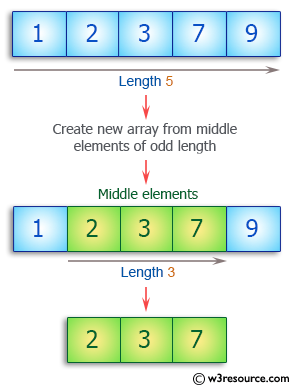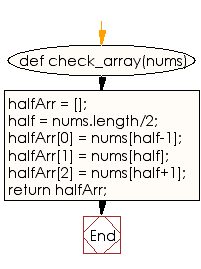﻿ Ruby Array exercises: Create a new array from the middle of a given array of integers of odd length - w3resource# Ruby Array Exercises: Create a new array from the middle of a given array of integers of odd length

## Ruby Array: Exercise-25 with Solution

Write a Ruby program to create a new array of length 3 containing the elements from the middle of a given array of integers of odd length (at least 3).Ruby Code:

``````def check_array(nums)
halfArr = [];
half = nums.length/2;
halfArr = nums[half-1];
halfArr = nums[half];
halfArr = nums[half+1];
return halfArr;
end

print check_array([1, 3, 4]),"\n"
print check_array([1, 2, 3, 7, 9])
``````

Output:

```[1, 3, 4]
[2, 3, 7]
```

Flowchart:Ruby Code Editor: